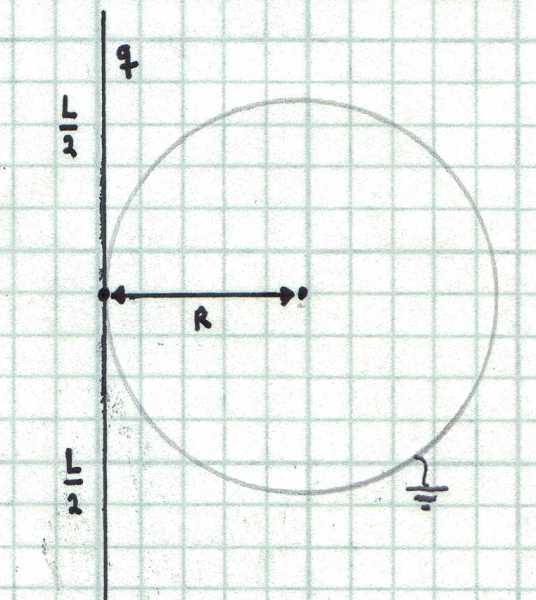# Determine The Induced ChargeConsider a dielectric thin rod with total charge $q=1~\mu\text{C}$ uniformly distributed over its length. The rod touches a grounded conducting sphere of radius $R=50~\text{cm}$ as shown in the figure below. Find the charged induced in the sphere in microcoulombs if the length of the rod is $L=1~\text{m}$. The following integral may be useful: $\int \frac{1}{\sqrt{a^{2}+x^{2}}}dx=\ln(x+\sqrt{x^2+a^{2}})+C.$

×